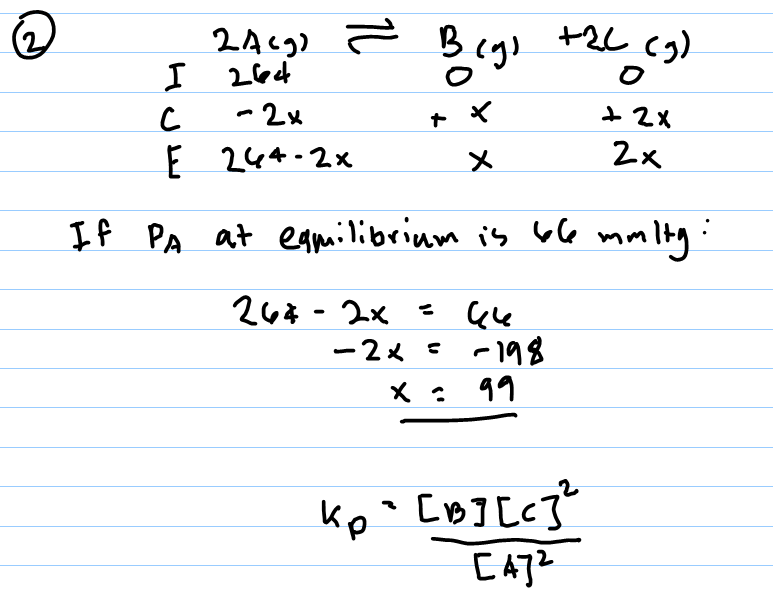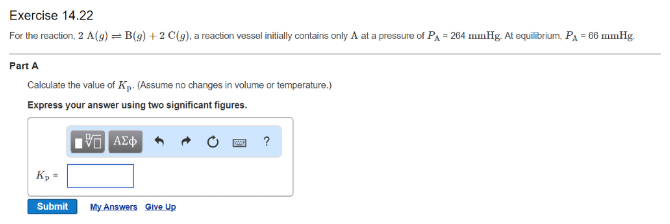# Problem: For the reaction, 2 A(g) ⇌ B(g) + 2 C(g), a reaction vessel initially contains only A at a pressure of PA = 264 mmHg. At equilibrium, PA = 66 mmHg. Calculate the value of K_p. (Assume no changes in volume or temperature.) Express your answer using two significant figures.

###### FREE Expert Solution88% (94 ratings)###### Problem Details

For the reaction, 2 A(g) ⇌ B(g) + 2 C(g), a reaction vessel initially contains only A at a pressure of PA = 264 mmHg. At equilibrium, PA = 66 mmHg. Calculate the value of K_p. (Assume no changes in volume or temperature.) Express your answer using two significant figures.